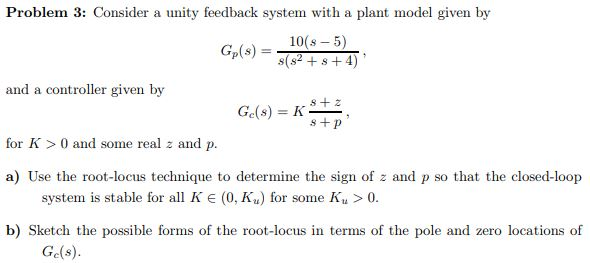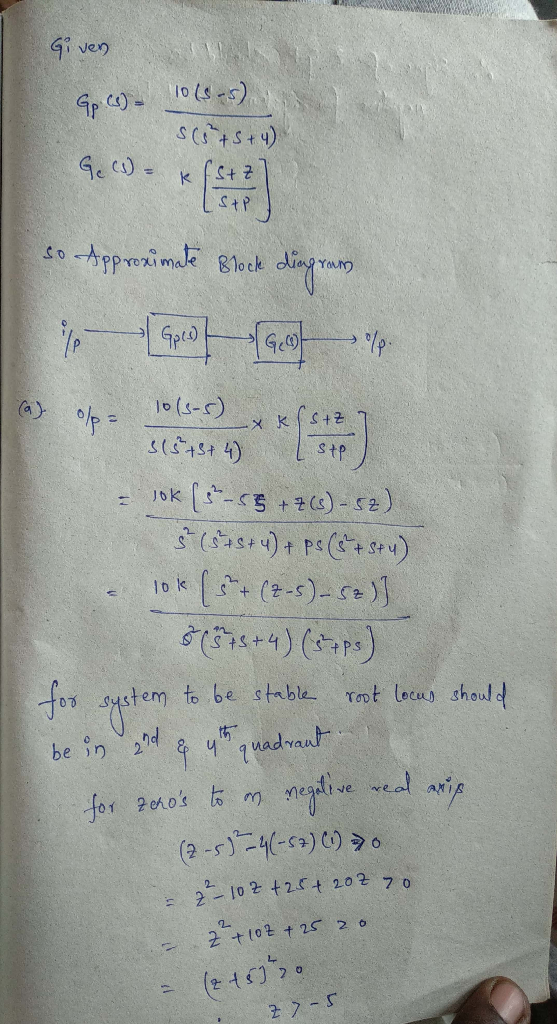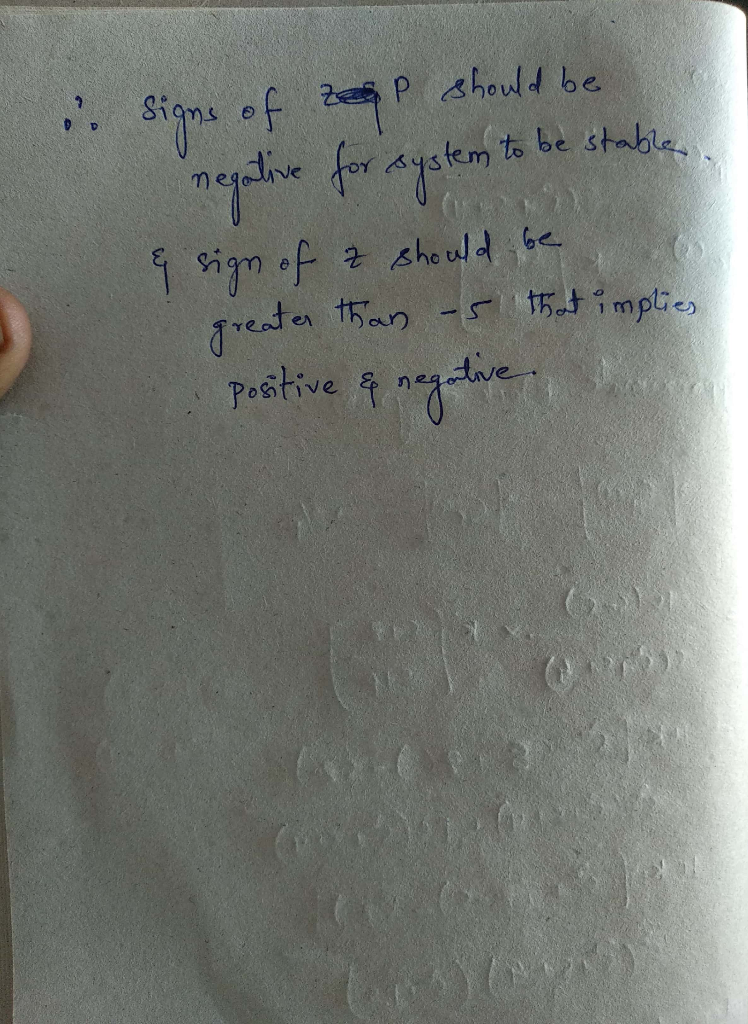Homework Help Question & Answers

# Problem 3: Consider a unity feedback system with a plant model given by 10(s- 5) and a controller...Problem 3: Consider a unity feedback system with a plant model given by 10(s- 5) and a controller given by s + p for K 0 and some real z and p. a) Use the root-locus technique to determine the sign of z and p so that the closed-loop system is stable for all K E (0, K) for some Ku> 0. b) Sketch the possible forms of the root-locus in terms of the pole and zero locations of Ge(s).

#### Homework Answers

ReportAnswer #1Add Homework Help Answer
##### Add Answer of: Problem 3: Consider a unity feedback system with a plant model given by 10(s- 5) and a controller...
Your Answer: Your Name: What's your source?
Not the answer you're looking for? Ask your own homework help question. Our experts will answer your question WITHIN MINUTES for Free.
More Homework Help Questions Additional questions in this topic.

• #### 1472) The plant of a magnetic-gap controller is: Gp(s)=1/((s+f)(s-f)). A controller Gc(s)=K(s+b)(...

Need Online Homework Help?

Get FREE EXPERT Answers
WITHIN MINUTES
Related Questions